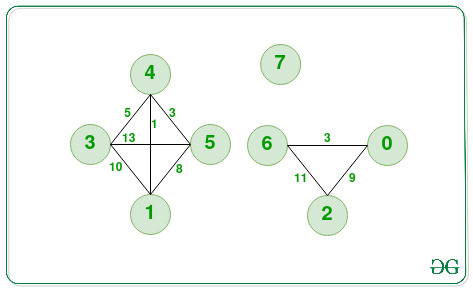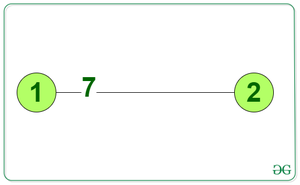# Find Maximum Shortest Distance in Each Component of a Graph

• Difficulty Level : Expert
• Last Updated : 21 Oct, 2021

Given an adjacency matrix graph[][] of a weighted graph consisting of N nodes and positive weights, the task for each connected component of the graph is to find the maximum among all possible shortest distances between every pair of nodes.

Examples:

Attention reader! Don’t stop learning now. Get hold of all the important DSA concepts with the DSA Self Paced Course at a student-friendly price and become industry ready.  To complete your preparation from learning a language to DS Algo and many more,  please refer Complete Interview Preparation Course.

In case you wish to attend live classes with experts, please refer DSA Live Classes for Working Professionals and Competitive Programming Live for Students.

Input:Output:

8 0 11

Explanation: There are three components in the graph namely a, b, c. In component (a) the shortest paths are following:

1. The shortest distance between 3 and 4 is 5 units.
2. The shortest distance between 3 and 1 is 1+5=6 units.
3. The shortest distance between 3 and 5 is 5+3=8 units.
4. The shortest distance between 1 and 4 is 1 unit.
5. The shortest distance between 1 and 5 is 1+3=4 units.
6. The shortest distance between 4 and 5 is 3 units.

Out of these shortest distances:
The maximum shortest distance in component (a) is 8 units between node 3 and node 5.
Similarly,
The maximum shortest distance in component (b) is 0 units.
The maximum shortest distance in component (c) is 11 units between nodes 2 and 6.

Input:Output:

Explanation: Since, there is only one component with 2 nodes having an edge between them of distance 7. Therefore, the answer will be 7.

Approach: This given problem can be solved by finding the connected components in the graph using DFS and store the components in a list of lists. Floyd Warshall’s Algorithm can be used to find all-pairs shortest paths in each connected component which is based on Dynamic Programming. After getting the shortest distances of all possible pairs in the graph, find the maximum shortest distances for each and every component in the graph. Follow the steps below to solve the problem:

• Define a function maxInThisComponent(vector<int> component, vector<vector<int>> graph) and perform the following steps:
• Initialize the variable maxDistance as INT_MIN and n as the size of the component.
• Iterate over the range [0, n) using the variable i and perform the following tasks:
• Iterate over the range [i+1, n) using the variable j and update the value of maxDistance as the maximum of maxDistance or graph[component[i]][component[j]].
• Return the value of maxDistance as the answer.
• Initialize a vector visited of size N and initialize the values as false.
• Initialize vectors, say components[][] and temp[] to store each component of the graph.
• Using Depth First Search(DFS) find all the components and store them in the vector components[][].
• Now, call the function floydWarshall(graph, V) to implement Floyd Warshall algorithm to find the shortest distance between all pairs of a component of a graph.
• Initialize a vector result[] to store the result.
• Initialize the variable numOfComp as the size of the vector components[][].
• Iterate over the range [0, numOfComp) using the variable i and call the function maxInThisComponent(components[i], graph) and store the value returned by it in the vector result[].
• After performing the above steps, print the values of the vector result[] as the answer.

Below is the implementation of the above approach:

## C++

 `// C++ program for the above approach``#include ``using` `namespace` `std;` `// Below dfs function will be used to``// get the connected components of a``// graph and stores all the connected``// nodes in the vector component``void` `dfs(``int` `src, vector<``bool``>& visited,``         ``vector >& graph,``         ``vector<``int``>& component, ``int` `N)``{` `    ``// Mark this vertex as visited``    ``visited[src] = ``true``;` `    ``// Put this node in component vector``    ``component.push_back(src);` `    ``// For all other vertices in graph``    ``for` `(``int` `dest = 0; dest < N; dest++) {` `        ``// If there is an edge between``        ``// src and dest i.e., the value``        ``// of graph[u][v]!=INT_MAX``        ``if` `(graph[src][dest] != INT_MAX) {` `            ``// If we haven't visited dest``            ``// then recursively apply``            ``// dfs on dest``            ``if` `(!visited[dest])``                ``dfs(dest, visited, graph,``                    ``component, N);``        ``}``    ``}``}` `// Below is the Floyd Warshall Algorithm``// which is based on Dynamic Programming``void` `floydWarshall(``    ``vector >& graph, ``int` `N)``{` `    ``// For every vertex of graph find``    ``// the shortest distance with``    ``// other vertices``    ``for` `(``int` `k = 0; k < N; k++) {``        ``for` `(``int` `i = 0; i < N; i++) {``            ``for` `(``int` `j = 0; j < N; j++) {` `                ``// Taking care of interger``                ``// overflow``                ``if` `(graph[i][k] != INT_MAX``                    ``&& graph[k][j] != INT_MAX) {` `                    ``// Update distance between``                    ``// vertex i and j if choosing``                    ``// k as an intermediate vertex``                    ``// make a shorter distance``                    ``if` `(graph[i][k] + graph[k][j]``                        ``< graph[i][j])``                        ``graph[i][j]``                            ``= graph[i][k] + graph[k][j];``                ``}``            ``}``        ``}``    ``}``}` `// Function to find the maximum shortest``// path distance in a component by checking``// the shortest distances between all``// possible pairs of nodes``int` `maxInThisComponent(vector<``int``>& component,``                       ``vector >& graph)``{``    ``int` `maxDistance = INT_MIN;``    ``int` `n = component.size();``    ``for` `(``int` `i = 0; i < n; i++) {``        ``for` `(``int` `j = i + 1; j < n; j++) {``            ``maxDistance``                ``= max(maxDistance,``                      ``graph[component[i]][component[j]]);``        ``}``    ``}` `    ``// If the maxDistance is still INT_MIN``    ``// then return 0 because this component``    ``// has a single element``    ``return` `(maxDistance == INT_MIN``                ``? 0``                ``: maxDistance);``}` `// Below function uses above two method``// to get the  maximum shortest distances``// in each component of the graph the``// function returns a vector, where each``// element denotes maximum shortest path``// distance for a component``vector<``int``> maximumShortesDistances(``    ``vector >& graph, ``int` `N)``{` `    ``// Find the connected components``    ``vector<``bool``> visited(N, ``false``);``    ``vector > components;` `    ``// For storing the nodes in a``    ``// particular component``    ``vector<``int``> temp;` `    ``// Now for each unvisited node run``    ``// the dfs to get the connected``    ``// component having this unvisited node``    ``for` `(``int` `i = 0; i < N; i++) {``        ``if` `(!visited[i]) {` `            ``// First of all clear the temp``            ``temp.clear();``            ``dfs(i, visited, graph, temp, N);``            ``components.push_back(temp);``        ``}``    ``}` `    ``// Now for all-pair find the shortest``    ``// path distances using Floyd Warshall``    ``floydWarshall(graph, N);` `    ``// Now for each component find the``    ``// maximum shortest distance and``    ``// store it in result``    ``vector<``int``> result;``    ``int` `numOfComp = components.size();``    ``int` `maxDistance;``    ``for` `(``int` `i = 0; i < numOfComp; i++) {``        ``maxDistance``            ``= maxInThisComponent(components[i], graph);``        ``result.push_back(maxDistance);``    ``}``    ``return` `result;``}` `// Driver Code``int` `main()``{``    ``int` `N = 8;``    ``const` `int` `inf = INT_MAX;` `    ``// Adjacency Matrix for the first``    ``// graph in the examples``    ``vector > graph1 = {``        ``{ 0, inf, 9, inf, inf, inf, 3, inf },``        ``{ inf, 0, inf, 10, 1, 8, inf, inf },``        ``{ 9, inf, 0, inf, inf, inf, 11, inf },``        ``{ inf, 10, inf, 0, 5, 13, inf, inf },``        ``{ inf, 1, inf, 5, 0, 3, inf, inf },``        ``{ 8, inf, inf, 13, 3, 0, inf, inf },``        ``{ 3, inf, 11, inf, inf, inf, 0, inf },``        ``{ inf, inf, inf, inf, inf, inf, inf, 0 },``    ``};` `    ``// Find the maximum shortest distances``    ``vector<``int``> result1``        ``= maximumShortesDistances(graph1, N);` `    ``// Printing the maximum shortest path``    ``// distances for each components``    ``for` `(``int` `mx1 : result1)``        ``cout << mx1 << ``' '``;` `    ``return` `0;``}`

## Javascript

 ``

Output
`11 8 0 `

Time Complexity: O(N3), where N is the number of vertices in the graph.
Auxiliary Space: O(N)

My Personal Notes arrow_drop_up